JAIC 1986, Volume 25, Number 1, Article 2 (pp. 15 to 29)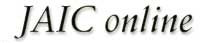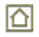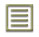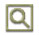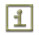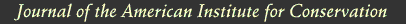JAIC 1986, Volume 25, Number 1, Article 2 (pp. 15 to 29)

### 6 DETERMINATION OF DIMER CHARGE

THE DETERMINATION of how much parylene dimer is needed to charge the system is based on the amount of surface area which is being coated in the depostion zone, and the coating thickness desired. The formula for making these determinations is based upon many years of practical experience in Nova Tran's deposition laboratories. However, most of this data is based upon work with smooth non-porous substrates such as metal, plastics, glass, etc. As discussed in the section on “Technical Problems,” paper contains large amounts of micro surface area. The very large surface areas contained in bound volumes necessitated some modification of existing formulae.

It was decided to prepare and test a group of several books in an effort to arrive at a new formula that would apply to bound volumes. The amount of apparent-surface area in each of the books was calculated and an arbitrary amount of parylene charge was assigned to each book.

The arbitrary formulae were as follows:

• Book #1 = .001 � apparent-surface area = � grams/dimer.
• Book #2 = .002 � apparent-surface area = � grams/dimer.
• Book #3 = .003 � apparent-surface area = � grams/dimer.
• Book #4 = .004 � apparent-surface area = � grams/dimer.
• Book #5 = .005 � apparent-surface area = � grams/dimer.
• Book #6 = .006 � apparent-surface area = � grams/dimer.

A total of 16 similar books from a series were parylene treated, three books at each level of treatment. Each of the three books at each level was treated with a different form or combination of forms of parylene. Parylene “C” and Parylene “N” are the most commonly used types, and of these “C” is used far more than “N.” Parylene “C” has slightly better moisture barrier properties and a faster deposition rate, while Parylene “N” has a slower deposition rate but greater penetration characteristics. This experiment would thus serve two purposes: (1) determine the correct dimer charge required for any given surface area, and (2) give some indication as to the best parylene type or combination of types which would give the best results.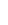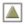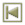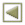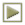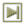Copyright � 1986 American Institute of Historic and Artistic Works